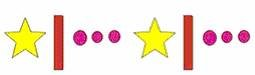## Definition Of Pattern

A Pattern constitutes a set of numbers or objects in which all the members are related with each other by a specific rule.

Pattern is also known as sequence.
There can be finite or infinite number of members in a pattern.

### Examples of PatternThe above pattern contains 2 identical groups with each group having 3 different images, a star followed by a bar, which is followed by 3 dots.

### Solved Example on Pattern

#### Ques: Find the next three terms of the following pattern. 81, 79, 77, ___, ___, ___,...

##### Choices:

A. 79, 81, and 83
B. 83, 81, and 79
C. 75, 73, and 71
D. 79, 77, and 75